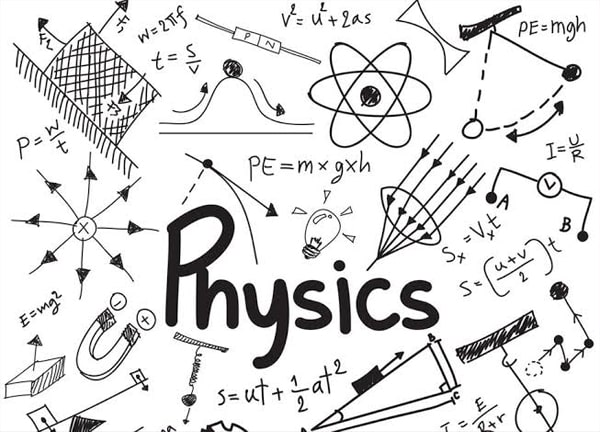Importance Of Physics in NEET 2020 Exam - Study24x7Default error msg

New to Study24x7 ?

# Importance Of Physics in NEET 2020 Exam

Updated on 15 February 2022NEET Preparation
Updated on 15 February 2022NEET full form is National Eligibility Cum Entrance Test which is conducted by CBSE for under-graduate admission in MBBS and BDS courses. Questions that come for this test is equally distributed from Class 11 and 12 studies.

Also, Physics in NEET is considered as one of the toughest subjects for many Biology based students. Therefore, for a good score in Physics, you must know chapter-wise weightage for NEET.

Though the NEET syllabus does not mention the weightage but through the previous year’s paper, you can gauge the weightage of a particular chapter. This is the way you can streamline and focus more on the NEET preparation.

so with this article you can analyse that Physics is quite important for NEET 2020 Exam

Based on previous year’s paper, here are some of the important chapters or topics which has more probability of high weightage in NEET 2020.## Kinematics:

Mechanics overall is the most important area for Physics NEET paper. Kinematics comes under Mechanics and deals with the study of motion. Average number of questions from this chapter is expected to be 2 that is 4% of the total questions of Mechanics.

Though the kinematics equation looks easy but students must not leave practicing as these are the areas where one can answer correctly and increase the score considerably.

Do not ignore the examples and numerical for NCERT textbooks. Try to consider real-life phenomena and relate problem. This would help you to draw diagrams and solve numerical. Be extremely hands-on with the vectors numerical.

Also, based on projectile motion practice numerical related to constrained motion or circular motion. This has importance not only in NEET but also in all types of All India JEE.

## Laws of Motion:

Learn the equation related to laws of motion and its use. Around 3 questions are expected from this part that is 7% of the total questions from Mechanics area. Practice as many numerical you can from this part.

## Work, Energy and Power:

‘Energy’ is a buzz word for most of the physics modules. Work, Energy and Power is a topic that comes under Mechanics and the average number of questions expected from this topic is 2 i.e 4% of the total questions of Mechanics.

Work energy theorem, equation related to gravitational potential energy are some of the areas that you need to concentrate as most of the questions appear from this part. Power is a smaller part so you can expect a theoretical question.

However, the students must also be thorough the theory apart from numerical. This is because at times real-life theoretical questions might become difficult to relate, if you are not thorough.

## Motion of System of Particles and Rigid Body:

You can expect around 3 questions from this module of Mechanics as well.

Mostly you can expect questions from the Centre of mass, Angular Momentum and Rotational motion of various objects. However, equations are very important to answer theoretical as well as numerical question from this topic.

Apart from the NCERT textbook, it is also advisable to practice from previous year papers for NEET. There are various institutions which would help you to gauge your stand and understand how much you are prepared for NEET 2020 Exam.

All The Best To All The NEET 2020 Aspirants!

Write a comment...Trending ArticlesBy CBSE CLASS 8By Edubabble LLPBy CBSE CLASS 7By CBSE CLASS 10
Related PostsServicesNeed Some Help!Connect